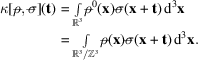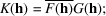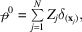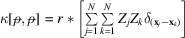International
Tables for
Crystallography
Volume B
Reciprocal space
Edited by U. Shmueli

International Tables for Crystallography (2010). Vol. B, ch. 1.3, pp. 64-65   | 1 | 2 |

## Section 1.3.4.2.1.6. Convolution, correlation and Patterson function

G. Bricognea

aGlobal Phasing Ltd, Sheraton House, Suites 14–16, Castle Park, Cambridge CB3 0AX, England, and LURE, Bâtiment 209D, Université Paris-Sud, 91405 Orsay, France

#### 1.3.4.2.1.6. Convolution, correlation and Patterson function

| top | pdf |

Letandbe two electron densities referred to crystallographic coordinates, with structure factorsand, so thatThe distributionis well defined, since the generalized support condition (Section 1.3.2.3.9.7) is satisfied. The forward version of the convolution theorem implies that ifthenIf eitheroris infinitely differentiable, then the distributionexists, and if we analyse it asthen the backward version of the convolution theorem reads:The cross correlationbetweenandis the-periodic distribution defined by:Ifandare locally integrable,LetThe combined use of the shift property and of the forward convolution theorem then gives immediately:hence the Fourier series representation of:Clearly,, as shown by the fact that permuting F and G changesinto its complex conjugate.

The auto-correlation ofis defined asand is called the Patterson function of. Ifconsists of point atoms, i.e.thencontains information about interatomic vectors. It has the Fourier series representationand is therefore calculable from the diffraction intensities alone. It was first proposed by Patterson (1934, 1935a,b) as an extension to crystals of the radially averaged correlation function used by Warren & Gingrich (1934)in the study of powders.

### References

Patterson, A. L. (1934). A Fourier series method for the determination of the components of interatomic distances in crystals. Phys. Rev. 46, 372–376.
Patterson, A. L. (1935a). A direct method for the determination of the components of interatomic distances in crystals. Z. Kristallogr. 90, 517–542.
Patterson, A. L. (1935b). Tabulated data for the seventeen plane groups. Z. Kristallogr. 90, 543–554.
Warren, B. E. & Gingrich, N. S. (1934). Fourier integral analysis of X-ray powder patterns. Phys. Rev. 46, 368–372.